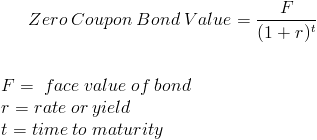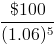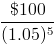Custom Search

# Zero Coupon Bond Value

Zero Coupon Bond PV Calculator (Click Here or Scroll Down)A zero coupon bond, sometimes referred to as a pure discount bond or simply discount bond, is a bond that does not pay coupon payments and instead pays one lump sum at maturity. The amount paid at maturity is called the face value. The term discount bond is used to reference how it is sold originally at a discount from its face value instead of standard pricing with periodic dividend payments as seen otherwise.

As shown in the formula, the value, and/or original price, of the zero coupon bond is discounted to present value. To find the zero coupon bond's value at its original price, the yield would be used in the formula. After the zero coupon bond is issued, the value may fluctuate as the current interest rates of the market may change.

### Example of Zero Coupon Bond Formula

A 5 year zero coupon bond is issued with a face value of \$100 and a rate of 6%. Looking at the formula, \$100 would be F, 6% would be r, and t would be 5 years.After solving the equation, the original price or value would be \$74.73. After 5 years, the bond could then be redeemed for the \$100 face value.

#### Example of Zero Coupon Bond Formula with Rate Changes

A 6 year bond was originally issued one year ago with a face value of \$100 and a rate of 6%. As the prior example shows, the value at the 6% rate with 5 years remaining would be \$74.73. In this example, we suppose that the interest rates have changed to 5% since it was originally issued.
The formula would be shown asAfter solving the equation, the value would be \$78.35.

New to Finance?